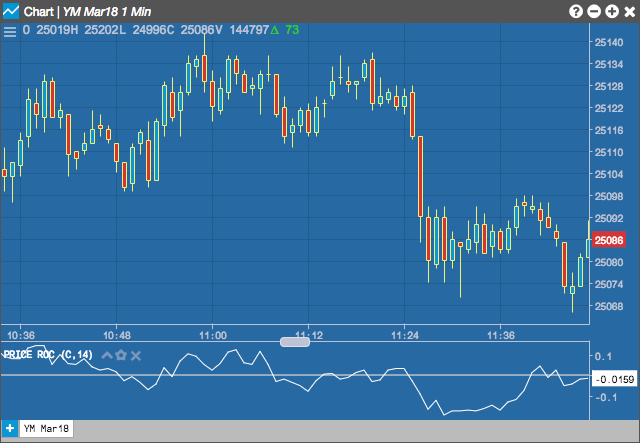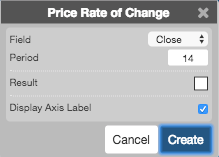Charts

# Price Rate of Change

The Price Rate of Change indicator is used to identify overbought or oversold conditions. It compares the current price with the previous price from a selected number of periods ago. The current price is divided by the previous price and expressed as a percentage. The rate increases until it reaches a market specific level. Extreme rates of increase could mean that the market is overheated and in need of a pause.## Configuration Options• Field: Price or combination of prices to use as the base for average calculations. Possible values include:
• Open
• High
• Low
• Close
• HL/2 $$\left ( \frac{High + Low}{2} \right )$$
• HLC/3 $$\left ( \frac{High + Low + Close}{3} \right )$$
• HLCC/4 $$\left ( \frac{High + Low + Close + Close}{4} \right )$$
• OHLC/4 $$\left ( \frac{Open + High + Low + Close}{4} \right )$$
$PROC = \frac{CurrentPrice - Price_{n-periods\ ago} * 100}{Price_{n-periods\ ago}} -1$